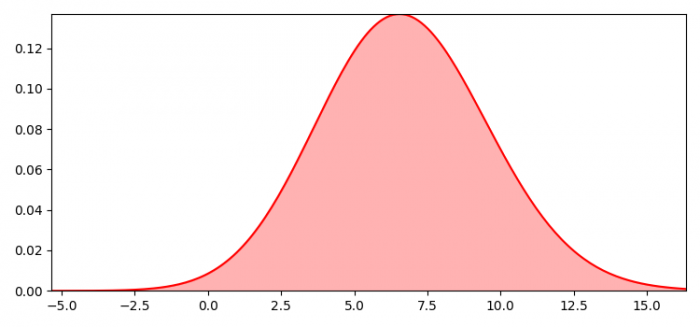# How to fill the area under a curve in a Seaborn distribution plot?

To fill the area under a curve in a Seaborn distribution plot, we can use distplot() and fill_between() methods.

## Steps

• Set the figure size and adjust the padding between and around the subplots.
• Create a list of data points.
• Plot a univariate distribution of observations.
• To fill the area under the curve, use fill_between() method.
• Set or retrieve autoscaling margins, x=0 and y=0.
• To display the figure, use show() method.

## Example

import seaborn as sns
import scipy.stats as stats
import matplotlib.pyplot as plt

plt.rcParams["figure.figsize"] = [7.50, 3.50]
plt.rcParams["figure.autolayout"] = True

x = [2.0, 7.5, 9.0, 8.5]
ax = sns.distplot(x, fit_kws={"color": "red"}, kde=False, fit=stats.gamma, hist=None, label="label 1")
l1 = ax.lines

x1 = l1.get_xydata()[:, 0]
y1 = l1.get_xydata()[:, 1]

ax.fill_between(x1, y1, color="red", alpha=0.3)
ax.margins(x=0, y=0)

plt.show()

## Output